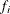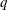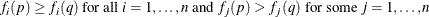### Optimizing Multiple Objectives

Many practical optimization problems involve more than one objective criteria, where the decision maker needs to examine trade-offs between conflicting objectives. With traditional optimization methods, these problems are often handled by aggregating multiple objectives into a single scalar objective, usually accomplished by some linear weighting of the multiple criteria. Other approaches involve turning objectives into constraints. One disadvantage of this strategy is that many separate optimizations with different weighting factors or constraints need to be performed to examine the trade-offs between different objectives. Genetic algorithms enable you to attack multiobjective problems directly, in order to evolve a set of solutions in one run of the optimization process instead of solving multiple separate problems.

This approach seeks to evolve the Pareto-optimal set: the set of solutions such that for each solution, all the objective criteria cannot be simultaneously improved. This is expressed mathematically by the concept of Pareto optimality. A Pareto-optimal set is the set of all nondominated solutions, according to the following definition of dominated:

For an-objective minimizing optimization problem, for each objective function, a solutionis dominated byifThe following is one strategy that can be employed in the GA procedure to evolve a set of Pareto-optimal solutions to a multiobjective optimization problem:

user objective function:

Define and specify a user objective function in a SetObjFunc call that computes each of the objective criteria and stores the objective values in one single solution segment.

user update routine:

Define and specify a user update routine in a SetUpdateRoutine call that examines the entire solution population and marks those in the Pareto-optimal set. This can be done with the MarkPareto call provided by the GA procedure. Also, set the elite parameter equal to the number of Pareto-optimal solutions found.

selection criteria:

Define a fitness comparison routine that favors the least dominated solutions, and designate it in a SetCompareRoutine call. For selecting between two solutions when neither solution dominates the other, your routine can check a secondary criterion to direct the search to the area of ultimate interest.

The multiple objective values are recorded in one segment to enable the use of the MarkPareto call provided by the GA procedure. Setting the elite selection parameter to the size of the Pareto-optimal set, in conjunction with the comparison criteria, guarantees that the Pareto-optimal set in each generation is preserved to the next.

The secondary comparison criterion can be used to ensure that the final Pareto-optimal set is distributed in the area of ultimate interest. For example, for the Bicriteria Constraint Strategy described previously, the actual area of interest is where there is zero constraint violation, which is the second objective. The secondary comparison criterion in that case is to minimize the value of the constraint violation objective. After enough iterations, the population should evolve to the point that the best solution to the bicriteria problem is also the best solution to the original constrained problem, and the other Pareto-optimal solutions can be examined to analyze the sensitivity of the optimum solution to the constraints. For other types of problems, you might need to implement a more complicated secondary comparison criterion to avoid crowding of solutions about some arbitrary point, and ensure the evolved Pareto-optimal set is distributed over a range of objective values.